A "Filled" Julia Set

The value of C in f(z)=z^2+C was chosen so that the following Julia Set would have an attracting 5-cycle. (See the Details section at the bottom for the actual value of C). The 5-cycle itself consists of the points A, B, C, D, and E; here f(A)=B, f(B)=C, f(C)=D, f(D)=E, and f(E)=A. We say informally that under iteration, "A goes to B, B goes to C, C goes to D, D goes to E and E goes to back to A. That this cycle is attracting means that under iteration, any other point in the black region sooner or later begins to generate a pattern that gets closer and closer to this star-shape. For example, in figure 2, the point a is in the black region (yes, it's hard to see, but it is!). This point a is sent to b under iteration, ie, f(a)=b. Then b is sent to c, c goes to d and so on. Eventually, the point m is reached, and once m goes to n, the sequence begins to look more like that star-shaped pattern in figure 1. The sequence of points a, b,c,... is called the orbit of a, and we say a is attracted to the 5-cycle.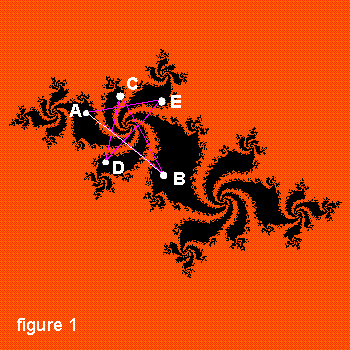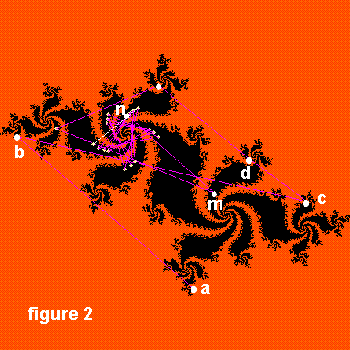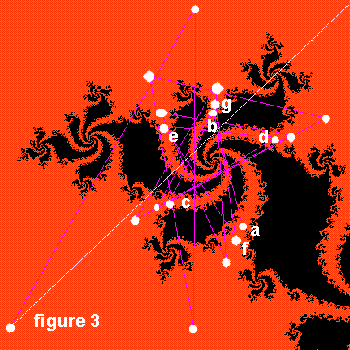Points in the orange region outside have orbits which move away, "out to infinity". In this figure, the point a is in the orange. a is sent to b (ie, f(a)=b), then b goes to c, and so on. Note that all the points in this orbit are in the orange, and they dance around, getting further away and then they're gone. We say the orbit of a "escapes to infinity". The Julia set itself is the boundary that separates the black from the orange regions. The orbit of any point on that boundary stays on it forever (although it's impossible to show this with computer programs since the exact coordinates of points on the boundary can't be stored --- to do so would require that the computer could store any and all numbers with "infinite precision"). Our computer program plots these orbits one point at a time dynamically; it's a fun and very educational process. Even more exciting is that as each point a, b, c... of the orbit is plotted, we can simultaneously play a tone whose pitch is dependent on the coordinates of the point, and in this way your ears can help you hear the periodicity of the attracting cycle as it is approached.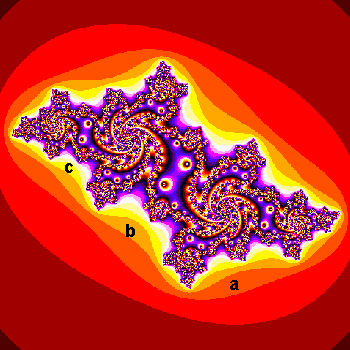Now, it's customary to color points in the plane according to how many iterates must be calculated before the long-term behavior of the points' orbits are determined. Members of any given orbit will eventually either (1) become big enough to indicate the orbit is "escaping to infinity", or (2) pass close enough to one of the members of the attractive 5-cycle to indicate the orbit is becoming attracted to it. In the following picture, it so happened that the orbits of all points in the orange region a all passed the "escape to infinity" test after just three members of their orbits were computed; all points in the region b took four iterations, and the points in the yellow region c took five iterations and so on. These colored bands are called level sets. The two "bullseyes" you see in the middle of the image surround points whose orbits very quickly became attracted to the 5-cycle. One of them surrounds the point B on the 5-cycle in figure 1, the other surrounds one of the two "preimages" of B; that is, it's a point B* satisfying f(B*)=B. (The other preimage of B is the point A on the 5-cycle). Again the bands of colors are called level sets.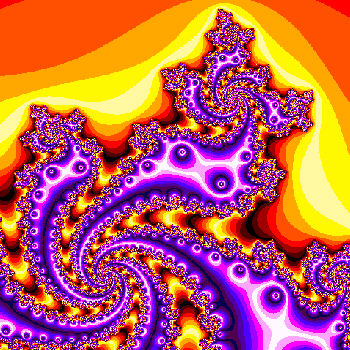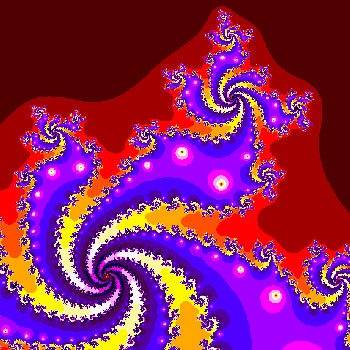The image above shows a zoom into our Julia set, and also displays a different coloring scheme: The red/yellow colors are reserved for the points whose orbits escape to infinity, and the blue/purple colors show points whose orbits become attracted to the 5-cycle. (Since we are using only 16 colors here, they must be "recycled" --- for example, points in yellow represent points whose orbits which escape at different numbers of iterations) This image shows the same portion of the Julia set as the one on the left, but the colors are changed according to the square root of the level set: Points which "escape" to infinity after 36-48 iterations get one color ("color number 6"), then 49-63 get color #7, 64--80 get color #8 and so on.

Our last image uses a 256-color scheme to display the same Julia set. See our fractal gallery (accessed from my home page) for other colorful fractals --- all using color schemes with important mathematical meanings.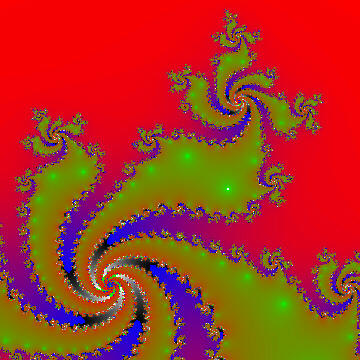Details: The value of C in f(z)=z^2+C used to produce this Julia set has real part -0.4701... and imaginary part 0.5664.... To know how to find such values to give you a Julia set with whatever number of points on the attractive cycle you want (5 in this case) involves knowing how to "read" the Mandlebrot set. It is possible to choose C so that the corresponding Julia set has no attracting cycles. See the Fractal Gallery for more examples.

In deciding whether an orbit was eventually "escaping to infinity", the computer used a test radius of 2, meaning if any point in an orbit was found to be more than 2 units from the origin, the orbit was declared as an escaping one.

In order to decide if an orbit was being attracted to the 5-cycle, the computer compared distances of points in the orbit to the point B on the attracting cycle. The test radius around B was 0.00001 units, meaning if an orbit member came within 0.00001 units of B, the orbit was declared as attracted to the 5-cycle. The real part of B is -0.09811... and the imaginary part was -0.08644...

Theses images were produced by an old-fashioned DOS program I wrote in Turbo/Borland Pascal, "screen-captured", and subsequently labeled using the Corel Print&Photo House programs.